# Count Birth Dates By Month in Excel

In this article, we will learn how to count birth dates from range by month Excel.

While working with dates, we may get the need to count the birth days which belong to the same month. In that case, this excel formula will easily let you know the count of birthdays, in a specific month.

How to solve the problem?

For this article we will use the SUMPRODUCT function. Here we are given birth dates in a range of data and specific monthly values. We need to count the date values where the formula includes all the date values of the given month.

Generic formula:

 = SUMPRODUCT(-- ( MONTH(range) = m_value ) )

range : birth date values given in as range

m_value : month number.

Example:

All of these might be confusing to understand. So, let's test this formula via running it on the example shown below.

Here we have the birth date records and we need to find the date values lying in a specific month or return the count rows where the date value lies within a given month number.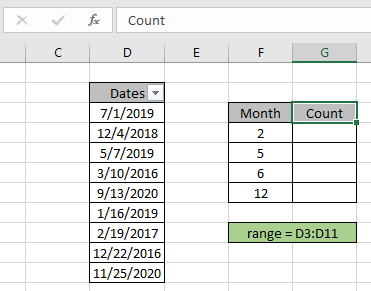Firstly, we need to define the named range for birthdates range as range. Just select the date values and type the name (range) for the range in the top left corner.

Now we will use the following formula to get a count of the dates which lay in the 2nd month which is February.
Use the Formula:

= SUMPRODUCT ( -- ( MONTH ( range ) = F4 ) )

range : named range used for birth date values D3:D11.

F4 : month value given as cell reference.

= : operator, condition given as equals to sign.

Explanation:

• MONTH ( range ) function extracts the monthly value from the birth date and matches it with the given month number and operator.

= SUMPRODUCT ( -- ( { 7 ; 12 ; 5 ; 3 ; 9 ; 1 ; 2 ; 12 ; 11 } = 2 ) )

• = operator matches it with month value 2 and returns an array TRUE and FALSE values depending on the operation.

= SUMPRODUCT ( -- { FALSE ; FALSE ; FALSE ; FALSE ; FALSE ; FALSE ; TRUE ; FALSE ; FALSE } )

• -- operator used to convert TRUE value to 1 & False value to 0.

= SUMPRODUCT ( { 0 ; 0 ; 0 ;  0 ; 0 ; 0 ; 1 ; 0 ; 0 } )

• SUMPRODUCT function gets the sum of 1s in the returned array, which will be the count of required birth dates.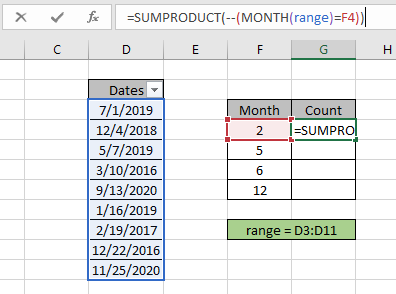Here the year value is given as cell reference and range is given as cell reference. Press Enter to get the count.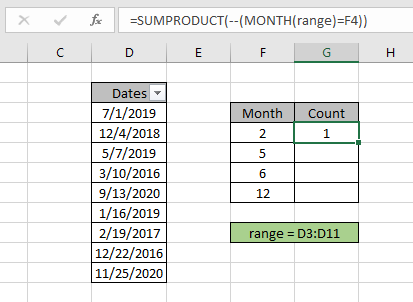As you can see the total date matches the 2 as month value comes out to be 1. It means there are 1 birthdate in the month of February.
Now copy and paste the formula to other cells using the CTRL + D or drag down option of Excel.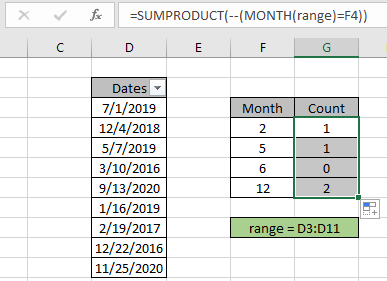As you can see in the above snapshot we obtain all the date values which lays in a specific year using the Excel formula. You can also obtain check the same using the excel filter option. Apply the filter to the Dates header and deselect the select all option.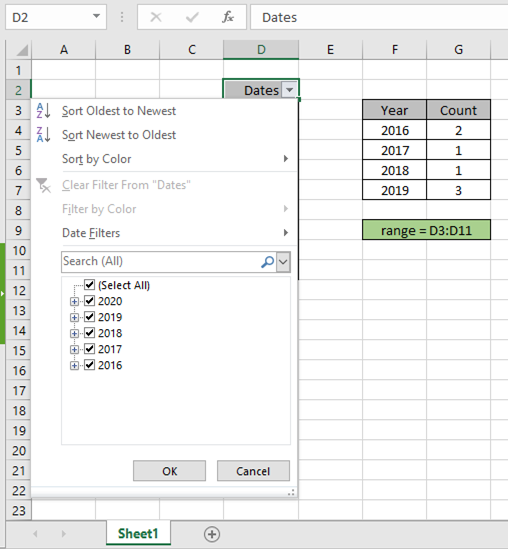For the month of February, select the Date filter option > All dates in this period > February to get the dates as shown below.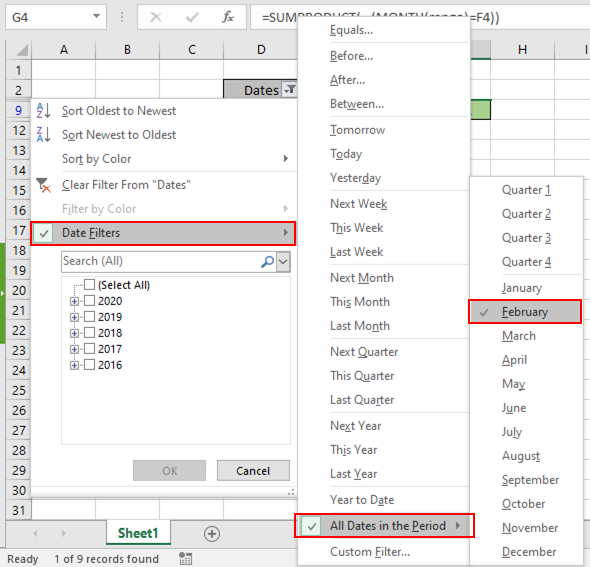After selecting the filtered dates by month, the dates will be like as shown in the snapshot below.

The filter option returns 1 value as result. Perform the same to other date values to get the count of dates or rows having criteria as given specific year.

Here are some observational notes shown below.
Notes:

1. The formula only works with numbers.
2. The SUMPRODUCT function considers non - numeric values as 0s.
3. The SUMPRODUCT function considers logic value TRUE as 1 and False as 0.
4. The argument array must be of the same length else the function.

Hope this article about how to Return Count if with SUMPRODUCT in Excel is explanatory. Find more articles on SUMPRODUCT functions here.

If you liked our blogs, share it with your friends on Facebook. And also you can follow us on Twitter and Facebook. We would love to hear from you, do let us know how we can improve, complement or innovate our work and make it better for you. Write us at info@exceltip.com

Related Articles

How to use the SUMPRODUCT function in Excel: Returns the SUM after multiplication of values in multiple arrays in excel.

COUNTIFS with Dynamic Criteria Range : Count cells dependent on other cell values in Excel.

COUNTIFS Two Criteria Match : Count cells matching two different criteria on list in excel.

COUNTIFS With OR For Multiple Criteria : Count cells having multiple criteria match using the OR function.

The COUNTIFS Function in Excel : Count cells dependent on other cell values.

How to Use Countif in VBA in Microsoft Excel : Count cells using Visual Basic for Applications code.

How to use wildcards in excel : Count cells matching phrases using the wildcards in excel

Popular Articles

50 Excel Shortcut to Increase Your Productivity

Edit a dropdown list

Absolute reference in Excel

If with conditional formatting

If with wildcards

Vlookup by date

Convert Inches To Feet and Inches in Excel 2016

Join first and last name in excel

Count cells which match either A or B

Terms and Conditions of use

The applications/code on this site are distributed as is and without warranties or liability. In no event shall the owner of the copyrights, or the authors of the applications/code be liable for any loss of profit, any problems or any damage resulting from the use or evaluation of the applications/code.